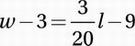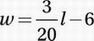# SAT Math Multiple Choice Question 744: Answer and Explanation

### Test Information

Question: 744

9.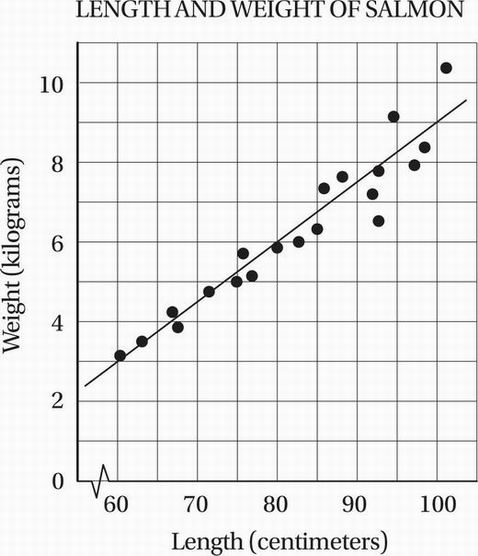Which of the following equations best describes the relationship between w, the weight in kilograms of each salmon, and l, its length in centimeters?

• A.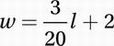• B.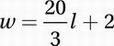• C.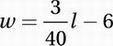• D.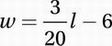Explanation:

D

Data Analysis (scatterplots) HARD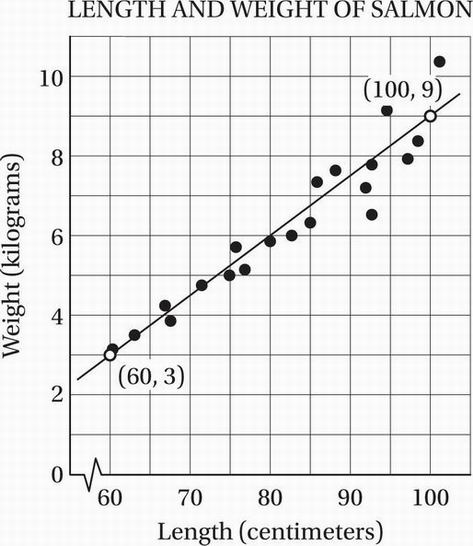To find the equation of the line of best fit, we can take two points on the line and then use the point-slope formula (Chapter 7, Lesson 5) to find the equation of the line. To get the most accurate representation of the line, we should choose two points that are fairly far apart, but whose coordinates are easy to determine. The graph shows that this line appears to pass through the points (60, 3) and (100, 9), and so, by the slope formula (Chapter 7, Lesson 5) we can calculate that the slope is (9 - 3)/(100 - 60) = 6/40 = 3/20. Using the first point in the

point-slope formula gives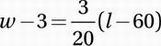Distribute: